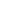# Free Polynomials Worksheets

Download free printable Polynomials Worksheets to practice. With thousands of questions available, you can generate as many Polynomials Worksheets as you want.

## Sample Polynomials Worksheet Questions

1.solve

1.

1/3

2.

-1/3

3.

2/3

4.

-2/3

2.

What is the degree of the following equation:

1.

1

2.

5

3.

4

4.

3

3.

How many real roots can a fourth degree polynomial have?

4.

What is an example of a 4th degree polynomial with exactly 4 terms?

5.

What is an example of a 5th degree polynomial with exactly 3 terms?

6.

What is an example of a 3rd degree polynomial?

7.

What is the degree of the polynomial 2x9 + 7x3 + 191?

8.

Choose the correct option: If 49x2 - b = (7x + 1/2)(7x - 1/2), then the value of b is ________.

1. 0 2. 3. 4.
9.

Choose the correct option: If p(x) = , then p() is equal to ________.

1. 0 2. 1 3. 4.
10.

Choose the correct option:  is a polynomial of degree ________.

1. 2 2. 0 3. 1 4. 1/2

Worksheets by UrbanPro

Our worksheets are designed to help students explore various topics, practice skills and enrich their subject knowledge, to improve their academic performance. Designed by Experts who have extensive experience and expertise in teaching a subject, these worksheets will improve your child's problem-solving skills and subject knowledge in a fun and interactive manner.
Check out our free customized worksheets across school boards, grades, subjects and levels of subject knowledge. You can download, print and share these worksheets with anyone, anywhere, anytime!

Get a custom worksheet to practice!

Select your topic & see the magic.

subjectSelect Chapter(s)

Chapters & Subtopics